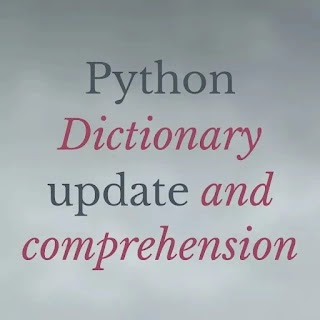# Python dictionary keys values method - codebugfreePython dictionary keys and value method

Python have the many datatypes. All datatypes are use the different purpose of work. Mainly use the dictionary datatypes. We discuss the Dictionary datatypes with his Key functio and method. Dictionary hava two two function. Key and Value pair. If you don't know the methos and the function of the key and value than you come a right place.

Python dictionary is the most use datatypes by a python programmer. Using of dictionary datatypes make a lot of python beginner and advance project like blood bank project. Meaning word dictionary. We already make the some project using the python datatypes.

SYNTAX

``````my_dict = {"name":"codebugfree","learn":"Dictionary_object"}
print(my_dict)``````

Here we will show the syntax of the dictionary. Name and learn is the key and codebugfree and dictionary object is the Value.

INOUTPUT

``{'name': 'codebugfree', 'learn': 'Dictionary_object'}``

Now we see the how to access only the key or value from the dictionary.

``````my_dict = {"name":"codebugfree","learn":"Dictionary_object"}
print(my_dict)
print(my_dict.keys())``````

It is the syntax of the key now we will see only the key from the my dict dictionary. See in the output

INOUTPUT

``````{'name': 'codebugfree', 'learn': 'Dictionary_object'}
dict_keys(['name', 'learn'])``````

When we use the keys method in dictionary name than print the key only from that dictionary. Length doesn't matter. If there is 100 key than all 100 key are print.

``````my_dict = {"name":"codebugfree","learn":"Dictionary_object"}
print(my_dict)
print(my_dict.values())``````

It is he syntax of the printing the key of the dictionary. When we use the values method in the dictionary name than print all the dictionary values.

INOUTPUT

``````my_dict = {"name":"codebugfree","learn":"Dictionary_object"}
print(my_dict)
print(my_dict.values())``````

If you don't know the how to update the dictionary than vist our python dictionary update post section. In that section we totally discuss the python dictionary method. Also their is example of dictionary method.